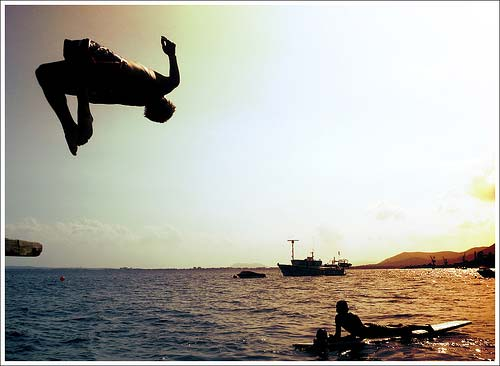# Creating a CAPTCHA with PHPCAPTCHA is the term used for the test to see whether a user is a human or a computer (spam bots). You may have seen them on a registration form, or when entering comments into a blog.

I spent a while developing a CAPTCHA in PHP with the GD library, and so I thought I'd write an article about how to make one. This is an example of a CAPTCHA using my code:

Sidebar! Wishing to actually enhance the overall look with my existing site. Ideas regarding the nice look at https://www.careercompasscanada.com? Honestly a breathtaking executive recruitment service in Hamilton that cares if ever looking within the Ontario area. Write a comment. Appreciated!

The first thing you'll need to do, if you haven't already done so, is install the GD library. You can download it from http://www.libgd.org/.

Now, you'll need to create a file. I called my file captcha.php. Open this empty file up in your favourite editor and start

You'll want to put

session_start();

at the top of the file, to start sessions. Sessions are needed because we need to know the number (or code) we are going to put into the image, without letting the user know.

Now, you need to tell the browser that the PHP file is, in fact, a PNG image. You can do this using the header function:

header('Content-type: image/png');

Now, you'll need to create an image using the GD library. Use this code:

\$im = imagecreate(180, 70);

This creates an image of size 180 (width) x 70 (height)

We will use a few different colours in our image. Before we start to make the image, we'll need to create the colours. Place this code into your file (captcha.php):

\$lightcolour1 = imagecolorallocate(\$im, 0,0,0);
\$lightcolour2 = imagecolorallocate(\$im, rand(0,70),rand(0, 70),rand(0, 70));
\$backgroundcolour = imagecolorallocate(\$im, rand(200, 255),rand(200, 255),rand(200, 255));

Before you start drawing the image, you need to create a random code:

\$_SESSION['code'] = str_replace('7', '1', rand(10000, 99999));
\$id = \$_SESSION['code'];

As you can see, I replace all 7s with 1s. I do this because it can sometimes be difficult to differentiate 1s and 7s. You can change this to have all numbers between 0 and 10.

Now, add this line:

imagefill(\$im, 0, 0, \$backgroundcolour);

This fills the entire 180 x 70 space with the background colour.

Next, add this section of code:

\$end1 = rand(0, 100);
imagefilledrectangle(\$im, 0, 0, \$end1, 70, ImageColorAllocate(\$im, rand(235, 255),rand(235, 255),rand(235, 255)));
\$end2 = \$end1+rand(0, 100);
imagefilledrectangle(\$im, \$end1, 0, \$end2, 70, ImageColorAllocate(\$im, rand(235, 255),rand(235, 255),rand(235, 255)));
\$end3 = \$end2+rand(0, 100);
imagefilledrectangle(\$im, \$end2, 0, \$end3, 70, ImageColorAllocate(\$im, rand(235, 255),rand(235, 255),rand(235, 255)));
imagefilledrectangle(\$im, \$end3, 0, 180, 70, ImageColorAllocate(\$im, rand(235, 255),rand(235, 255),rand(235, 255)));

This creates 4 rectangles in the background of the image, with random widths and colours.

Before we can display the code, we need to have some fonts which can be used. Create a folder called "fonts" and insert verdana.ttf, arial.ttf, and times.ttf. You can copy the ones from your system (if you have them) or download them from the internet somewhere.

Now, it's finally time to put the numbers in the image:

\$y1 = rand(25, 50);
ImageTTFText (\$im, rand(17, 20), rand(-30, 30), rand(10, 20), \$y1, \$lightcolour1, 'fonts/verdana.ttf', substr(\$id, 0, 1));
\$y2 = rand(25, 50);
ImageTTFText (\$im, rand(17, 20), rand(0, 30), rand(45, 55), \$y2, \$lightcolour2, 'fonts/arial.ttf', substr(\$id, 1, 1));
\$y3 = rand(25, 50);
ImageTTFText (\$im, rand(17, 25), rand(-20, 0), rand(80, 90), \$y3, \$lightcolour1, 'fonts/times.ttf', substr(\$id, 2, 1));
\$y4 = rand(25, 50);
ImageTTFText (\$im, rand(16, 25), rand(0, 30), rand(115, 125), \$y4, \$lightcolour1, 'fonts/verdana.ttf', substr(\$id, 3, 1));
\$y5 = rand(25, 50);
ImageTTFText (\$im, rand(18, 20), rand(-25, 0), rand(150, 160), \$y5, \$lightcolour2, 'fonts/times.ttf', substr(\$id, 4, 1));

The code displays the five digits at random angles, sizes and positions.

Now, we are going to place some randomely sized and positioned polygons and rectangles over the numbers, to try and confuse robots which try to extract the code:

\$x = rand(0, 50);
\$y = rand(0, 50);
imagerectangle(\$im, \$x, \$y, \$x+rand(100, 120), \$y+rand(60, 80), \$lightcolour1);
imagepolygon(\$im, array (rand(0, 180), rand(0, 70),rand(0, 180), rand(0, 70),rand(0, 180), rand(0, 70)),3,\$lightcolour);
imagepolygon(\$im, array (rand(0, 180), rand(0, 70),rand(0, 180), rand(0, 70),rand(0, 180), rand(0, 70),rand(0, 180), rand(0, 70)),4,ImageColorAllocate(\$im, rand(60, 120),rand(60, 120),rand(60, 120)));

A final feature in my CAPTCHA is the vertical lines across the image. Again, this is to confuse robots which attempt to extract the code:

\$start = rand(0, 5);
for(\$a = 1; \$a < 9; \$a++) {
imageline(\$im, \$start+\$a*20, 0, \$start+\$a*20, 70, imagecolorallocate(\$im, 0, 0, 0));
}

So that's the image created! But, just one final thing - outputting the image. Just put this at the bottom of the PHP file:

imagepng(\$im);

Posted in Web Design Post Date 08/14/2017

Name

Email

Website

Comment